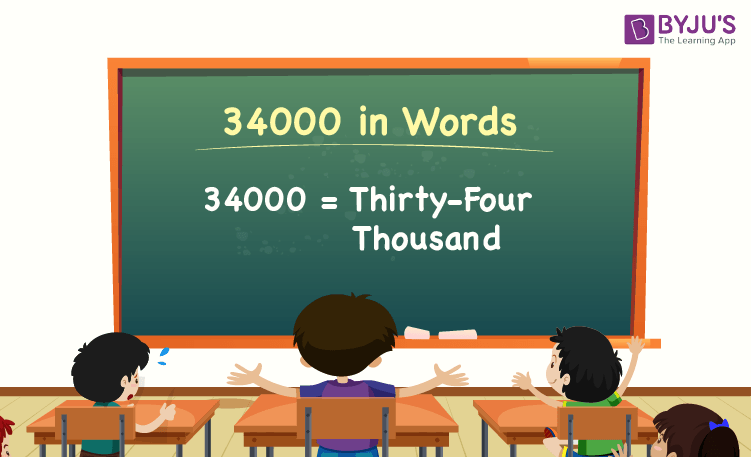# 34000 in Words

34000 in words is Thirty-four Thousand. For example, if you spent Rs. 34000 in three months, you can write, “I have spent Rs. Thirty-four Thousand in three months”. The number name of 34000 can be written using the place value of a number.

 34000 in Words: Thirty-four Thousand

In this article, we will discuss how to write 34000 in words, and look at the solved examples in detail.

## How to Write 34000 in Words?To write the number name of 34000, first, determine the place value of each digit of the given number 34000. Hence, for the number 34000, the digit in one’s place is 0, the digit in ten’s place is 0, the digit in hundred’s place is 0, and the digit in thousand’s place is 34.

For the number 34000,

1’s place of 34000 is 0

10’s place of 34000 is 0.

100’s place of 34000 is 0.

1000’s place of 34000 is 34.

Thus, the place value chart for 34000 is:

 Thousands Hundreds Tens Ones 34 0 0 0

Therefore, the number 34000 in words is Thirty-four Thousand.

### Examples

Example 1:

Find the value of 14000 + 20000. Describe the value in words.

Solution:

Given expression: 14000 + 20000

⇒ 14000 + 20000 = 34000

So, the value of 14000 + 20000 is 34000.

Hence, 34000 in words is Thirty-four Thousand.

Example 2:

Express the value of thirty-four thousand minus ten thousand in words.

Solution:

Thirty-four Thousand = 34000

Ten thousand = 10000

Thirty-four Thousand minus ten thousand = 34000 – 10000 = 24000

Hence, the value of thirty-four thousand minus ten thousand is twenty-four thousand in words.

## Frequently Asked Questions on 34000 in Words

Q1

### Write 34000 in words.

34000 in words is thirty-four thousand.

Q2

### Express the value of 31000 + 3000 in words.

Simplifying 31000 + 3000, we get 34000. Hence, 34000 in words is thirty-four thousand.

Q3

### How to write thirty-four thousand in numbers?

Thirty-four thousand in numbers is 34000.

Q4

### Is the number 34000 an even number?

Yes, the number 34000 is an even number.

Q5

### Is 34000 a perfect square number?

No, 34000 is not a perfect square number.